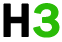C++基础 专题

C++ 函数

c++ 标准库提供了大量的程序可以调用的内置函数。例如，函数 strcat() 用来连接两个字符串，函数 memcpy() 用来复制内存到另一个位置。

## 定义函数

c++ 中的函数定义的一般形式如下：

```return_type function_name( parameter list )
{
body of the function
}
```

• 返回类型：一个函数可以返回一个值。return_type 是函数返回的值的数据类型。有些函数执行所需的操作而不返回值，在这种情况下，return_type 是关键字 void
• 函数名称：这是函数的实际名称。函数名和参数列表一起构成了函数签名。
• 参数：参数就像是占位符。当函数被调用时，您向参数传递一个值，这个值被称为实际参数。参数列表包括函数参数的类型、顺序、数量。参数是可选的，也就是说，函数可能不包含参数。
• 函数主体：函数主体包含一组定义函数执行任务的语句。

## 实例

``````// 函数返回两个数中较大的那个数

int max(int num1, int num2)
{
// 局部变量声明
int result;

if (num1 > num2)
result = num1;
else
result = num2;

return result;
}
``````

## 函数声明

```return_type function_name( parameter list );
```

```int max(int num1, int num2);
```

```int max(int, int);
```

## 调用函数

``````#include <iostream>
using namespace std;

// 函数声明
int max(int num1, int num2);

int main ()
{
// 局部变量声明
int a = 100;
int b = 200;
int ret;

// 调用函数来获取最大值
ret = max(a, b);

cout << "max value is : " << ret << endl;
return 0; }   // 函数返回两个数中较大的那个数
int max(int num1, int num2)  {    // 局部变量声明
int result;
if (num1 > num2)
result = num1;
else
result = num2;

return result;
}
``````

```max value is : 200
```

## 参数的默认值

``````#include <iostream>
using namespace std;

int sum(int a, int b=20)
{
int result;

result = a + b;

return (result);
}

int main ()
{
// 局部变量声明
int a = 100;
int b = 200;
int result;

// 调用函数来添加值
result = sum(a, b);
cout << "total value is :" << result << endl;     // 再次调用函数
result = sum(a);
cout << "total value is :" << result << endl;
return 0;
} ``````

```total value is :300
total value is :120
```